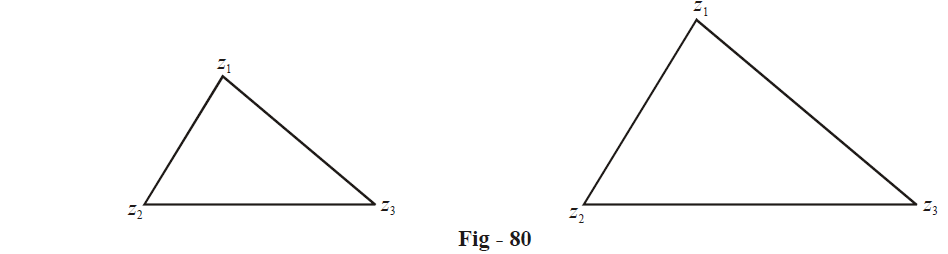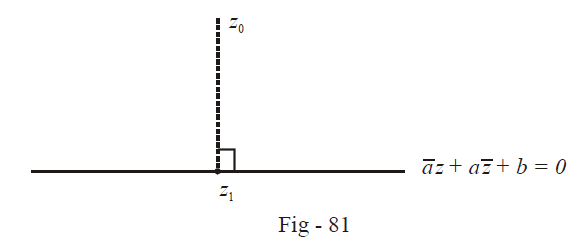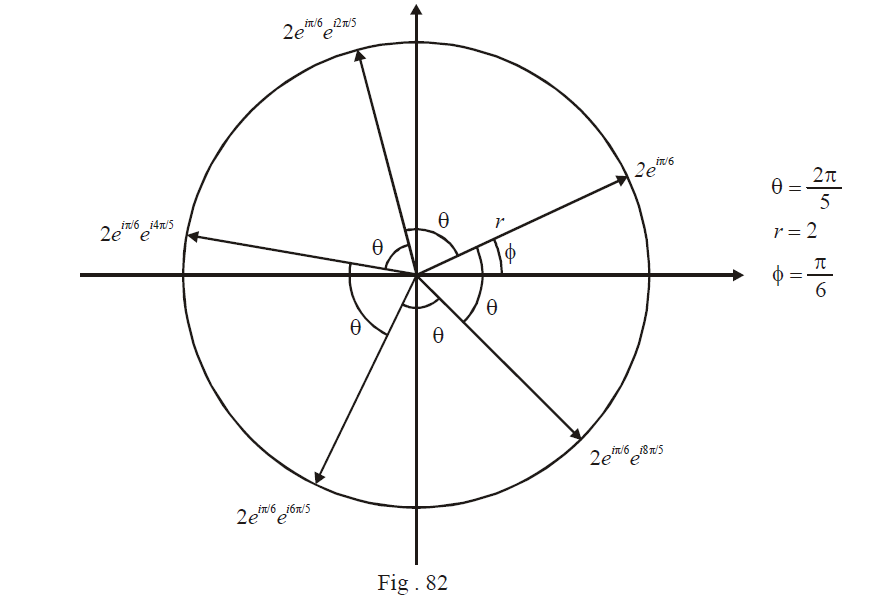# Complex Numbers Set 5

Go back to  'SOLVED EXAMPLES'

Example- 17

Show that the triangles whose vertices are $${z_1},\,\,{z_2},\,\,{z_3}{\text{ and }}{Z_1},\,\,{Z_2},\,\,{Z_3}$$  are directly similar if

$\left| {\begin{array}{*{20}{c}}{{z_1\;\;}}{{\;Z_1\;\;}}1\\{{z_2\;\;}}{{Z_2\;\;}}1\\{{z_3\;\;}}{{Z_3\;\;}}1\end{array}} \right| = 0$

Solution:Since the triangles are directly similar, the vector $${z_1} - {z_2}$$  will be a scalar multiple of $${Z_1} - {Z_2};$$ the vector $${z_2} - {z_3}$$  will be the (same) scalar multiple of $${Z_2} - {Z_3}$$ and so on:

\begin{align}&\qquad{z_1} - {z_2} = \lambda ({Z_1} - {Z_2})\\&\qquad{z_2} - {z_3} = \lambda ({Z_2} - {Z_3})\\ &\Rightarrow \,\,\,\frac{{{z_1} - {z_2}}}{{{z_2} - {z_3}}} = \frac{{{Z_1} - {Z_2}}}{{{Z_2} - {Z_3}}}\\&\Rightarrow \,\,\,{z_1}({Z_2} - {Z_3}) + {z_2}({Z_3} - {Z_1}) + {z_3}({Z_1} - {Z_2}) = 0\end{align}\\\!\!\!\!\!\!\!\!\!\!\!\!\!\!\!\!\!\!\!\!\!\!\!\!\!\!\!\!\!\!\!\!\!\!\!\Rightarrow \,\,\,\left| {\begin{gathered}{{z_1}}{{\;\;\;Z_1}}\;\;1\\{{\;\;\;z_2}}{{\;\;\;Z_2}}\;\;\;1\\{{\;\;\;z_3\;\;}}{{\;\;\;Z_3\;\;}}1\end{gathered}} \right| = 0

Example- 18

Show that the perpendicular distance of a point $${{z}_{0}}$$from the line $$\bar az + a\bar z + b = 0$$ $$(b \in \mathbb{R})$$is

$\frac{{\left| {a{{\bar z}_0} + \bar a{z_0} + b} \right|}}{{2\left| a \right|}}$

Solution:Let $${z_1}$$ be the foot of the perpendicular dropped from $${z_0}$$ onto the given line. We need to evaluate $$\left| {{z_0} - {z_1}} \right|$$  .

Now, since $${z_1}$$ lies on the given line, we have

$\bar a{z_1} + a{\bar z_1} + b = 0\;\;\;\;\;\; \;\;\;\;... (1)$

Also, the complex slopes of the given line and the perpendicular must add to 0:

\begin{align}&\quad\qquad\frac{{ - a}}{{\bar a}} + \frac{{{z_0} - {z_1}}}{{{{\bar z}_0} - {{\bar z}_1}}} = 0\\ &\Rightarrow \,\,\, - a({{\bar z}_0} - {{\bar z}_1}) + \bar a({z_0} - {z_1}) = 0\\ &\Rightarrow \,\,\, - a{{\bar z}_0} + \bar a{z_0} + a{{\bar z}_1} - \bar a{z_1} = 0 \;\;\;\;\;\; \;\;\;\;... (2)\end{align}

From (1) + (2),

\begin{align}&\qquad - a{{\bar z}_0} + \bar a{z_0} + 2a{{\bar z}_1} + b = 0\\ &\Rightarrow \,\,\,{{\bar z}_1} = \frac{{a{{\bar z}_0} - \bar a{z_0} - b}}{{2a}}\\ &\Rightarrow \,\,\,{{\bar z}_1} - {{\bar z}_0} = \frac{{ - a{{\bar z}_0} - \bar a{z_0} - b}}{{2a}}\\ &\Rightarrow \,\,\,\left| {{z_1} - {z_0}} \right| = \frac{{\left| {a{{\bar z}_0} + \bar a{z_0} + b} \right|}}{{2\left| a \right|}}\end{align}

Example- 19

Find all  $$a\in \mathbb{R}$$  if these exists one z which satisfies $$\left| z \right| = 3,\,\,\left| {z - (a(1 + i) - i)\,} \right| \le 3$$and $$\left| {z + 2a - (a + 1)i} \right| > 3$$ simultaneously.

Solution:  Since $$\left| z \right| = 3,\,\,z$$  must lie on a circle of radius 3 centred at the origin. Now, the distance of z from a(1 + i) – i must not be greater than 3, i.e., z must lie inside a circle of radius 3 centred at  a(1 + i) – i. Thus, the |z| = 3 circle and the latter circle must intersect (or at least touch) in order that both the relations |z| = 3 and $$\left| {z - (a(1 + i) - 1)} \right| \le 3$$   are  satisfied. This means that the distance between the centres of the two circles must be less than the sum of the radii, i.e., 6

\begin{align}{} \Rightarrow \,\,\,\left| {a(1 + i) - 1} \right| \le 6\\\\ \Rightarrow \,\,\,\left| {(a - 1) + ai} \right| \le 6\\\\ \Rightarrow \,\,\,{(a - 1)^2} + {a^2} \le 36\\\\ \Rightarrow \,\,\,2{a^2} - 2a - 35 \le 0\\\\ \Rightarrow \,\,\,\frac{{1 - \sqrt {71} }}{2} \le a \le \frac{{1 + \sqrt {71} }}{2} \;\;\;\;\; \;\;\;\;\; \ldots (1)\end{align}

By an analogous argument, for the relation $$\left| {z + 2a - (a + 1)i} \right| > 3$$ to be satisfied simultaneously, z must lie outside a circle of radius 3 centred at $$\left| {z + 2a - (a + 1)i} \right| > 3$$. Thus, the distance between the two centres of the two circles $$\left| z \right| = 3$$ and this circle must be greater than the sum of the radii.

\begin{align}{} \Rightarrow \,\,\,\left| {2a - (a + 1)i} \right| > 6\\\\ \Rightarrow \,\,\,5{a^2} + 2a + 1 > 36\\\\ \Rightarrow \,\,\,a < \frac{{ - 1 - 4\sqrt {11} }}{5}{\rm{ or }}a > \frac{{ - 1 + 4\sqrt {11} }}{5} \ldots (2)\end{align}

The intersection of (1) and (2) gives

$\left[ {\frac{{1 - \sqrt {71} }}{2},\,\,\frac{{ - 1 - 4\sqrt {11} }}{5}} \right) \cup \left( {\frac{{ - 1 + 4\sqrt {11} }}{5},\,\,\frac{{1 + \sqrt {71} }}{2}} \right]$

Example- 20

Plot the fifth roots $$16( - \sqrt 3 + i)$$  of  on the plane

Solution:   We first write $$z = 16( - \sqrt 3 + i)$$ in its Euler form.

\begin{align}&z = 16( - \sqrt 3 + i) = 32{e^{i5\,\pi /6}}\\\\&\;\;=32{{e}^{i\left( 2p\pi +\frac{5\pi }{6} \right)}},p\in \mathbb{Z}\\\\&\Rightarrow \,\,\,{{z}^{1/5}}=2{{e}^{i\left( \frac{2p\pi }{5}+\frac{\pi }{6} \right)}},\,\,\,\,p\in \mathbb{Z}\end{align}

To obtain the roots, we let p take on five consecutive integral values, say p = 0, 1, 2, 3, 4. The roots obtained are:

$2{e^{i\pi /6}},2{e^{i\pi /6}}{e^{i2\pi /5}},2{e^{i\pi /6}}{e^{i4\pi /5}},2{e^{i\pi /6}}{e^{i6\pi /5}},2{e^{i\pi /6}}{e^{i8\pi /5}}$

These five roots will lie evenly spaced out at angles of 72° between any two consecutive roots. The first root is at an angle of 30°.Learn from the best math teachers and top your exams

• Live one on one classroom and doubt clearing
• Practice worksheets in and after class for conceptual clarity
• Personalized curriculum to keep up with school